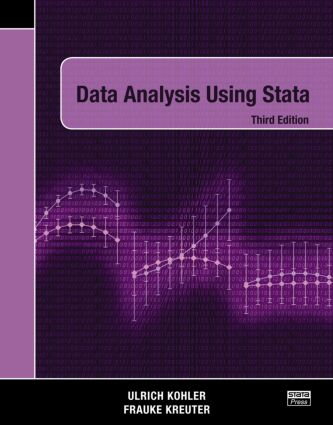# Data Analysis Using Stata, Third Edition

## 3rd Edition

Stata Press

497 pages | 22 B/W Illus.

Paperback: 9781597181105
pub: 2012-08-20
\$88.95
x

FREE Standard Shipping!

### Description

Data Analysis Using Stata, Third Edition is a comprehensive introduction to both statistical methods and Stata. Beginners will learn the logic of data analysis and interpretation and easily become self-sufficient data analysts. Readers already familiar with Stata will find it an enjoyable resource for picking up new tips and tricks.

The book is written as a self-study tutorial and organized around examples. It interactively introduces statistical techniques such as data exploration, description, and regression techniques for continuous and binary dependent variables. Step by step, readers move through the entire process of data analysis and in doing so learn the principles of Stata, data manipulation, graphical representation, and programs to automate repetitive tasks. This third edition includes advanced topics, such as factor-variables notation, average marginal effects, standard errors in complex survey, and multiple imputation in a way, that beginners of both data analysis and Stata can understand.

Using data from a longitudinal study of private households, the authors provide examples from the social sciences that are relatable to researchers from all disciplines. The examples emphasize good statistical practice and reproducible research. Readers are encouraged to download the companion package of datasets to replicate the examples as they work through the book. Each chapter ends with exercises to consolidate acquired skills.

The First Time

Starting Stata

Do-files

Exiting Stata

Working with Do-Files

From interactive work to working with a do-file

Designing do-files

The Grammar of Stata

The elements of Stata commands

Repeating similar commands

Weights

General Comments on the Statistical Commands

Regular statistical commands

Estimation commands

Creating and Changing Variables

The commands generate and replace

Specialized recoding commands

Recoding string variables

Recoding date and time

Setting missing values

Labels

Storage types, or the ghost in the machine

Creating and Changing Graphs

A primer on graph syntax

Graph types

Graph elements

Multiple graphs

Saving and printing graphs

Describing and Comparing Distributions

Categories: Few or many?

Variables with few categories

Variables with many categories

Statistical Inference

Random samples and sampling distributions

Descriptive inference

Causal inference

Introduction to Linear Regression

Simple linear regression

Multiple regression

Regression diagnostics

Model extensions

Reporting regression results

Regression Models for Categorical Dependent Variables

The linear probability model

Basic concepts

Logistic regression with Stata

Logistic regression diagnostics

Likelihood-ratio test

Refined models

The goal: The data matrix

Inputting data

Combining data

Saving and exporting data

Handling big datasets

Do-Files for Advanced Users and User-Written Programs

Two examples of usage

Four programming tools

User-written Stata commands

Around Stata

Resources and information

Taking care of Stata

References

Author Index

Subject Index

Exercises appear at the end of each chapter.